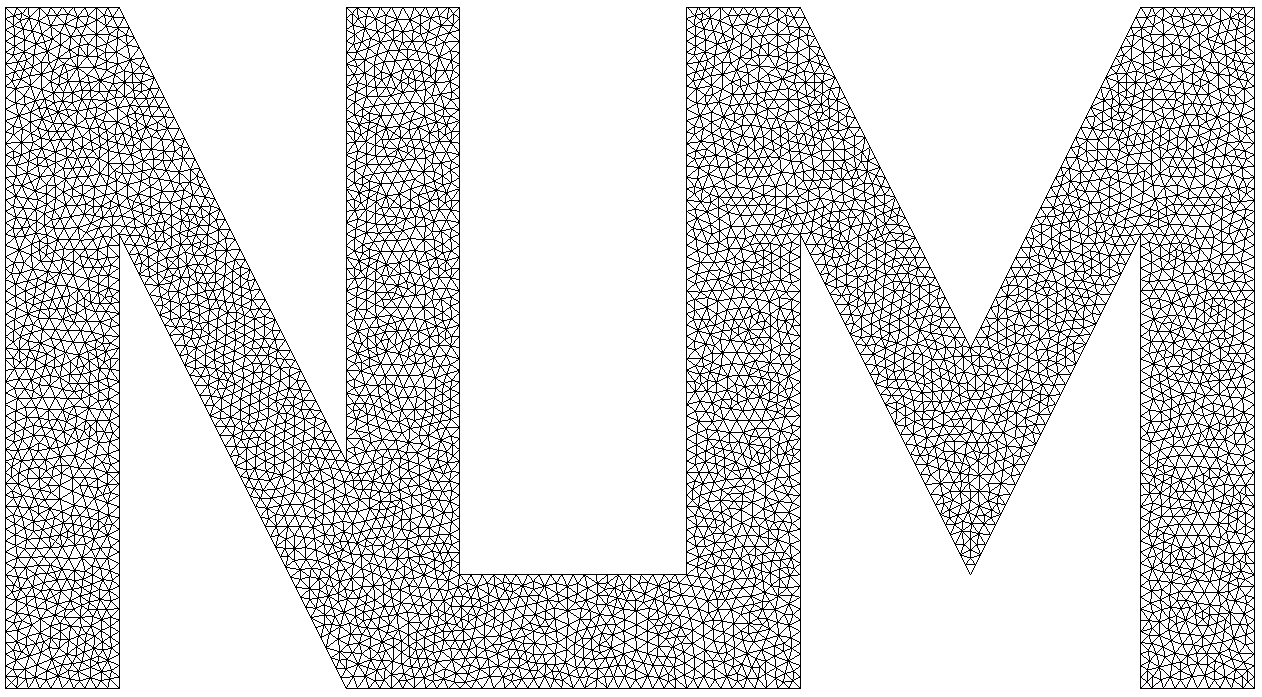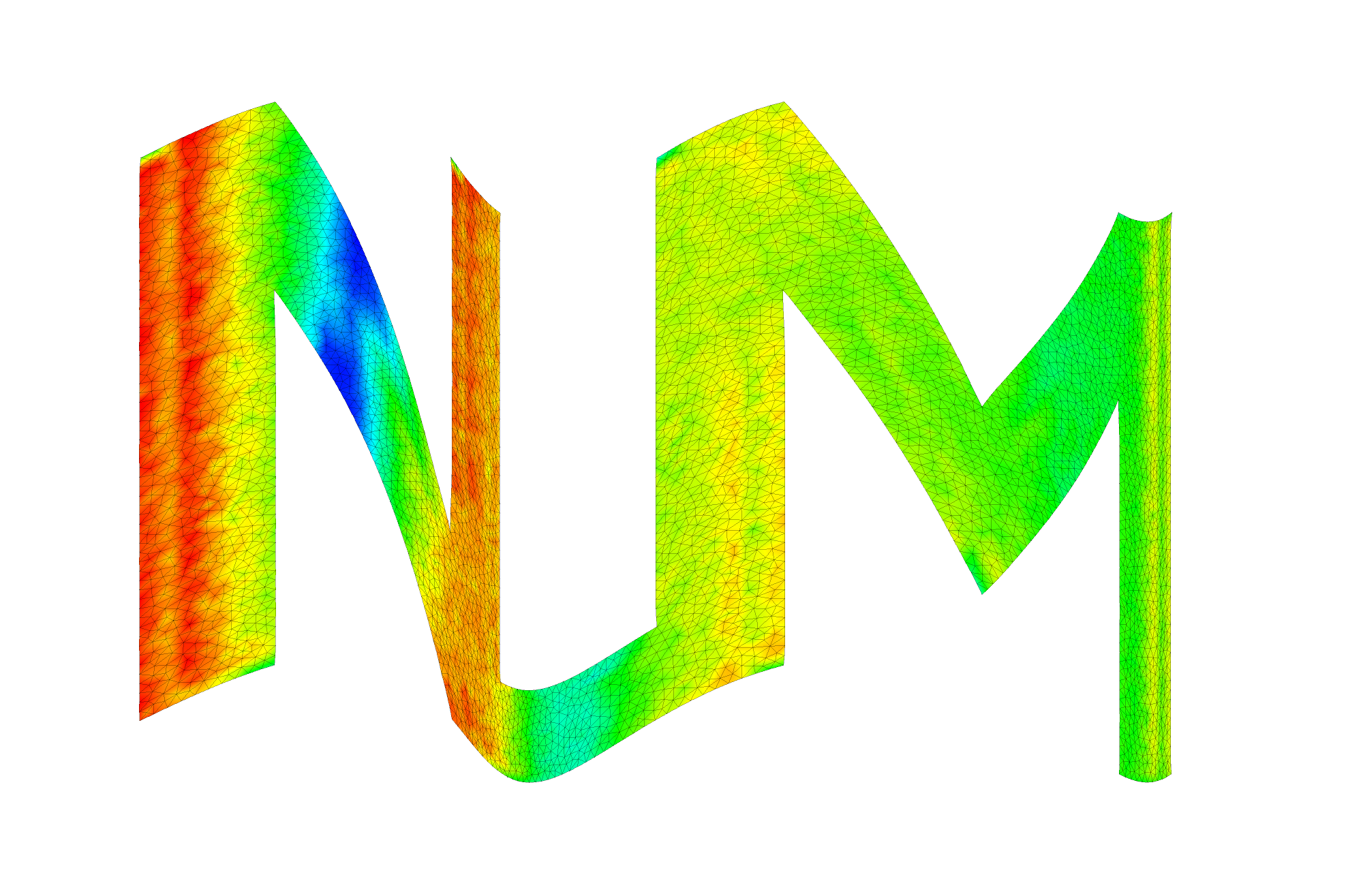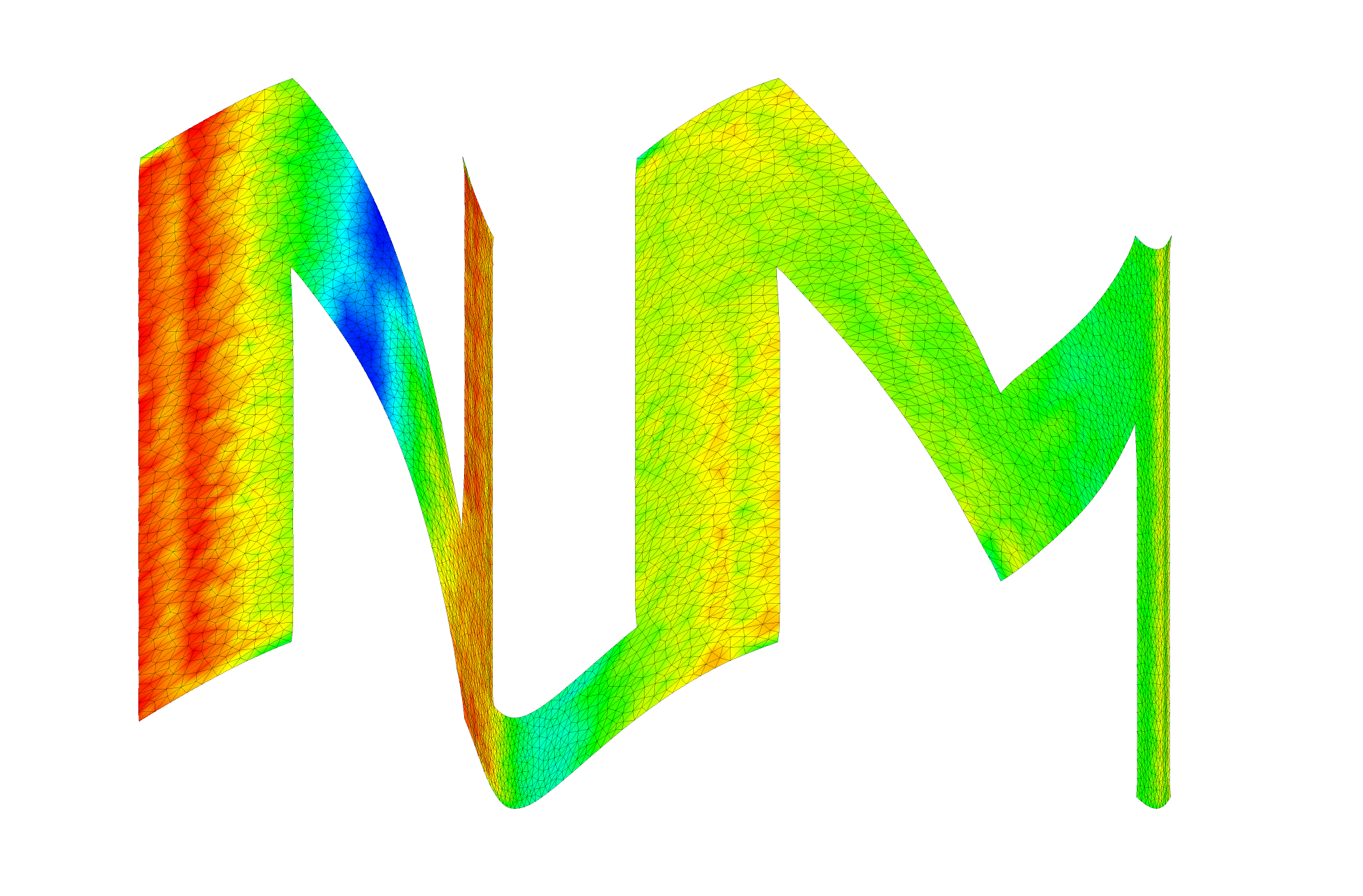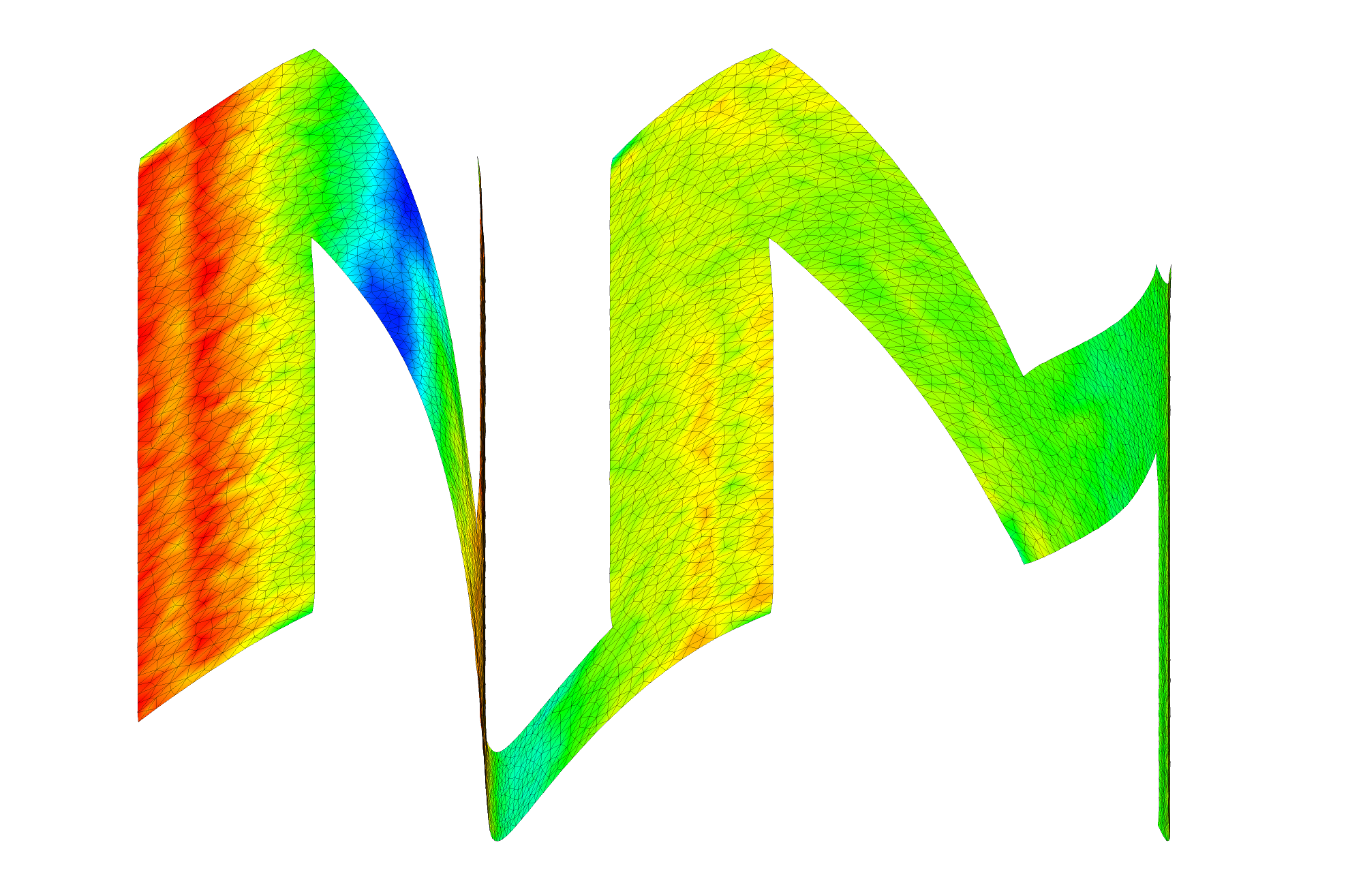• The setup is same as in the first Logo example except the boundary condition.

• Boundary condition

• We apply body force along horizontal axis on whole material domain.

• Force is given by $f_x(x,y,t) = f t sin(n\pi x/L_x)$ where n = 3 and f = 1.5E+09.

• We apply body force along vertical axis on whole material domain.

• Force is given by $f_y(x,y,t) = f t sin(n\pi y/L_y)$ where n = 2 and f = 1.5E+09.

• We specify Displacement, Velocity, Force and Damage_Z as output candidates.

### Mesh

We obtain mesh using Gmsh library with mesh size h = 0.025.### Results

We show damage plot at time t = 0.008, 0.009, 0.01 seconds.Video of simulation: